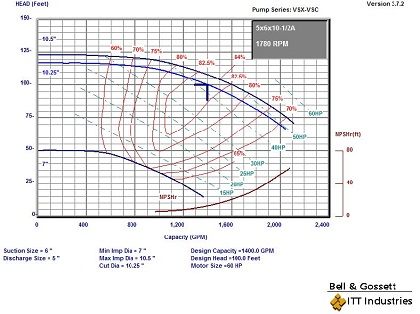# Heat Added to Chilled Water Systems Due to Pump Efficiency

Norm Hall
/
August 9, 2010
Printer Friendly (PDF)

 We received an interesting question this week from a consultant engineer: “How much temperature increase will I get in the chilled water from the pump? My secondary chilled water pump has a capacity of 1400 GPM at 100 feet” Let’s look at the answer. ANSWER: Centrifugal pumps in hydronic systems do the work of moving gallons per minute of fluid against a differential head in feet. This work is defined as Brake Horsepower (BHP) also expressed in equation 1. The maximum amount of horsepower available to heat the water is 1 minus the pump efficiency multiplied by the motor horsepower. Bell and Gossett refers to this as the Heating Horsepower (HHP) expressed in equation 2. 1 HP equals 42.44 BTU/Minute (equation 3) and BTU/minute is GPM times ∆T times 8.33 times specific gravity times specific heat (equation 4). The later two items are equal to 1 for water. Using all of this we arrive at a formula for ∆T shown as equation 5.BHP= (GPM X Ft hd)/ (3960 X Eff)     – Equation 1 HHP= BHP X (1-Eff)                            – Equation 2 1 HP= 42.44 BTU/M                           – Equation 3 BTU/M = GPM X ∆T X 8.33 X 1 X 1   – Equation 4 ∆T = (42.44 X HHP)/ (8.33 X GPM)  – Equation 5 ∆T = (5.09 X HHP)/ GPM                    – Equation 6 Let’s look at the example requested by the engineer. We selected a 60 HP pump using the ITT Bell and Gossett ESP-PLUS program. The BHP, using equation 1, is (1400 X 100) / (3960 X .84) = 42.09 HP. At the design point we have an efficiency of 84% so HHP is 42.09 X .16 or 6.73 HP and ∆T is (5.09 X 6.73)/1400 or .02 degrees F. We can answer the question with the response, “Not very much”!Disclaimer: R. L. Deppmann and it’s affiliates can not be held liable for issues caused by use of the information on this page. While the information comes from many years of experience and can be a valuable tool, it may not take into account special circumstances in your system and we therefore can not take responsibility for actions that result from this information. Please feel free to contact us if you do have any questions.